## Geometric Series

It's almost the last section, Shmoopers. A geometric series is just the added-together version of a geometric sequence. Like this:

1 + 2 + 4 + 8 + 16 + 32 + ...

Instead of just listing all the terms with commas in between, we take the sum of everything.

We use the same sigma notation we used with arithmetic series, so we have a general form that looks like this: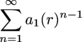In this series, our numbers will start when n = 1 and go all the way to infinity. Geometric series are unique in this way. Not only can we find partial sums like we did with arithmetic sequences, we can find the overall sum as well. We'll do both; cool your jets.

Before we jump into sample problems, we'll need two formulas to find these sums. The first is the formula for the sum of an infinite geometric series.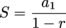This formula really couldn't be much simpler. All we need is the first term and the common ratio and boom—we have the sum.

Unfortunately, and this is a big "unfortunately," this formula will only work when we have what's known as a convergent geometric series. A convergent series is one whose partial sums get closer to a certain value as the number of terms increases. We think this will help…

The series with the terms 1, 2, 4, 8, 16, 32, ... does not converge because every time we put another number into our sum, the sum gets a lot bigger. We can see that each time we add in another number, the sum is going to get larger and larger and larger and larger and larger and larger…you get the idea. This series is divergent, not convergent.

On the other hand, the series with the terms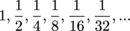has a sum that also increases with each additional term. However, each time we add in another term, the sum is not going to get that much bigger. This is especially true when we add in terms like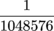. This is only the 21st term of this series, but it's very small.

While the ideas of convergence and divergence are a little more involved than this, for now, this working knowledge will do. In fact, we can tell if an infinite geometric series converges based simply on the value of r.

When |r| < 1, the series converges.

When |r| ≥ 1, the series diverges.

This means it only makes sense to find sums for the convergent series since divergent ones have sums that are infinitely large. This is true even though the formula we gave you technically gives you a number when you put in a1 and r, even for divergent series.

The other formula is for a finite geometric series, which we use when we only want the sum of a certain number of terms. The nth partial sum of a geometric series is given by: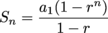It simply means that we're only going to add up the first n terms. That might be 5, 10, or 20 terms. The bottom line is we're not adding them all the way to infinity.

This formula is really close to our original formula. The only difference is the (1 – rn). It works for any geometric series regardless of the value of r.

Let's see these guys in action.

### Sample Problem

Find the sum of the infinite geometric series:

3 + 6 + 12 + 24 + ...

Nice try. In this series, r = 2. In other words, we're doubling each term, so our common ratio is 2. Last time we checked, 2 > 1. That means the series diverges and its sum is infinitely large. So we can't find the sum.

### Sample Problem

Find the sum of the infinite geometric series given by: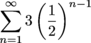.

Much better. This one's a convergent series with a first term of a1 = 3 and a common ratio of r = ½. We'll use our formula and then get on with our lives.There it is. Even though we're adding an infinite number of terms, their sum will get closer and closer to 6.

### Sample Problem

Find the fifth partial sum of the geometric series:

2 + 6 + 18 + ...

We're multiplying each term by 3, so our common ratio is 3. Let's take the partial sum formula and substitute a1 = 2 and r = 3. We can also put in 5 for n since we're asked to find the fifth partial sum.Notice our answer here is big. But that's kind of expected since this series would be considered a divergent infinite geometric series and, as a result, has some seriously big partial sums along the way.

### Sample Problem

Find the sum: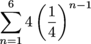.

Formula to the rescue…again. Note that n will be 6 in our formula since our series is set up to go from n = 1 to n = 6.It sure isn't pretty. But it works.

Nice job. That's a wrap.

## This is a premium product